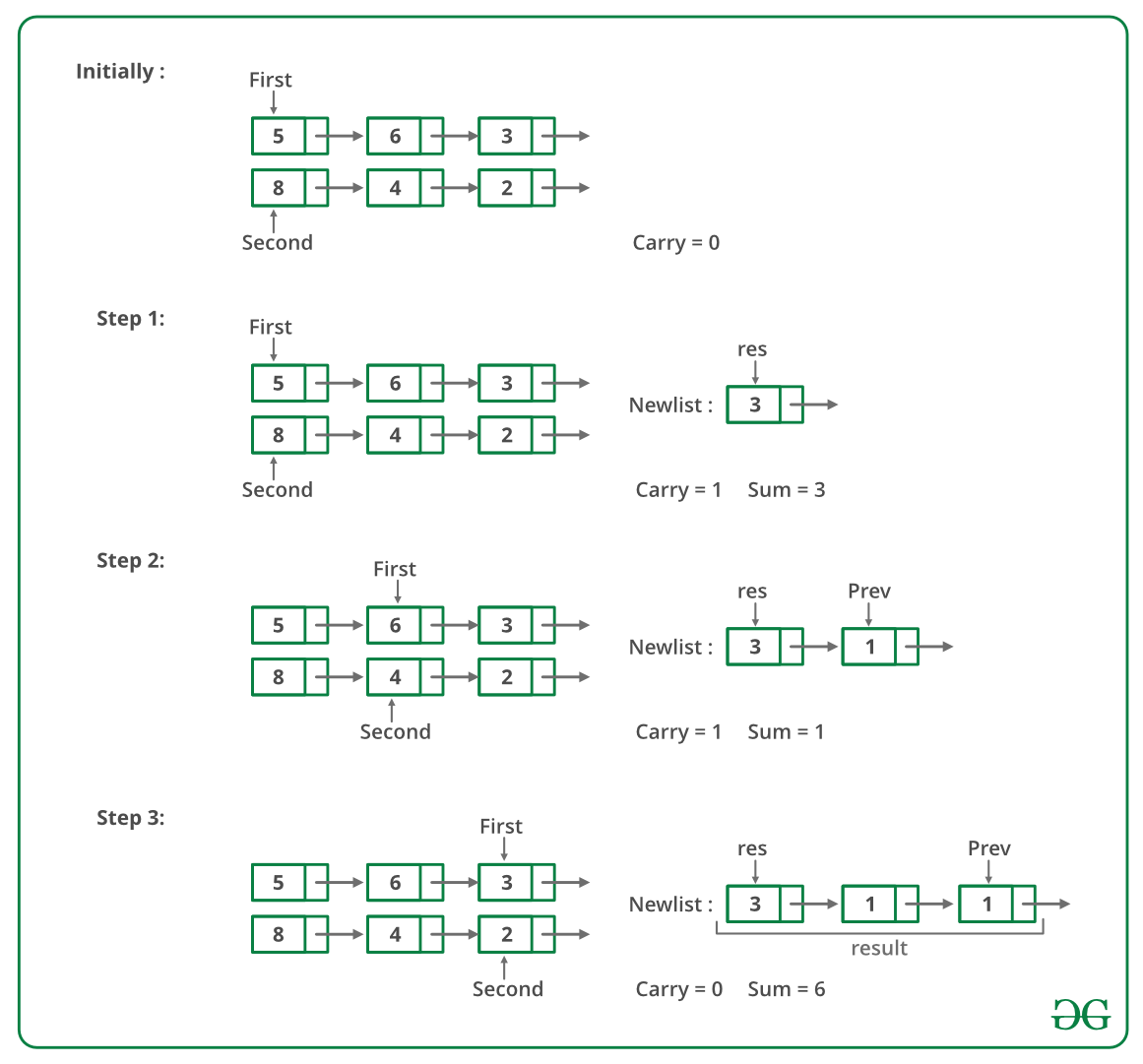GeeksforGeeks App
Open AppBrowser
Continue

# C Program For Adding Two Numbers Represented By Linked Lists- Set 2

Given two numbers represented by two linked lists, write a function that returns the sum list. The sum list is linked list representation of the addition of two input numbers. It is not allowed to modify the lists. Also, not allowed to use explicit extra space (Hint: Use Recursion).

Example :

```Input:
First List: 5->6->3
Second List: 8->4->2

Output:
Resultant list: 1->4->0->5```

We have discussed a solution here which is for linked lists where a least significant digit is the first node of lists and the most significant digit is the last node. In this problem, the most significant node is the first node and the least significant digit is the last node and we are not allowed to modify the lists. Recursion is used here to calculate sum from right to left.

Following are the steps.
1) Calculate sizes of given two linked lists.
2) If sizes are same, then calculate sum using recursion. Hold all nodes in recursion call stack till the rightmost node, calculate the sum of rightmost nodes and forward carry to the left side.
3) If size is not same, then follow below steps:
….a) Calculate difference of sizes of two linked lists. Let the difference be diff
….b) Move diff nodes ahead in the bigger linked list. Now use step 2 to calculate the sum of the smaller list and right sub-list (of the same size) of a larger list. Also, store the carry of this sum.
….c) Calculate the sum of the carry (calculated in the previous step) with the remaining left sub-list of a larger list. Nodes of this sum are added at the beginning of the sum list obtained the previous step.

Below is a dry run of the above approach:Below image is the implementation of the above approach.

## C

 `// A C recursive program to add two``// linked lists``#include ``#include ` `// A linked List Node``struct` `Node``{``    ``int` `data;``    ``struct` `Node* next;``};` `typedef` `struct` `Node node;` `/* A utility function to insert a``   ``node at the beginning of``   ``linked list */``void` `push(``struct` `Node** head_ref,``          ``int` `new_data)``{``    ``// Allocate node``    ``struct` `Node* new_node =``           ``(``struct` `Node*)``malloc``(``sizeof``(``struct` `Node));` `    ``// Put in the data ``    ``new_node->data = new_data;` `    ``// Link the old list off the``    ``// new node``    ``new_node->next = (*head_ref);` `    ``// Move the head to point to the``    ``// new node``    ``(*head_ref) = new_node;``}` `// A utility function to print``// linked list``void` `printList(``struct` `Node* node)``{``    ``while` `(node != NULL)``    ``{``        ``printf``(``"%d  "``, node->data);``        ``node = node->next;``    ``}``    ``printf``(``"n"``);``}` `// A utility function to swap``// two pointers``void` `swapPointer(Node** a, Node** b)``{``    ``node* t = *a;``    ``*a = *b;``    ``*b = t;``}` `/* A utility function to get size``   ``of linked list */``int` `getSize(``struct` `Node* node)``{``    ``int` `size = 0;``    ``while` `(node != NULL)``    ``{``        ``node = node->next;``        ``size++;``    ``}``    ``return` `size;``}` `// Adds two linked lists of same``// size represented by head1``// and head2 and returns head of``// the resultant linked list.``// Carry is propagated while``// returning from the recursion``node* addSameSize(Node* head1,``                  ``Node* head2,  ``                  ``int``* carry)``{``    ``// Since the function assumes``    ``// linked lists are of same``    ``// size, check any of the two``    ``// head pointers``    ``if` `(head1 == NULL)``        ``return` `NULL;` `    ``int` `sum;` `    ``// Allocate memory for sum``    ``// node of current two nodes``    ``Node* result =``          ``(Node*)``malloc``(``sizeof``(Node));` `    ``// Recursively add remaining nodes``    ``// and get the carry``    ``result->next = addSameSize(head1->next,``                               ``head2->next, carry);` `    ``// Add digits of current nodes``    ``// and propagated carry``    ``sum = head1->data + head2->data + *carry;``    ``*carry = sum / 10;``    ``sum = sum % 10;` `    ``// Assign the sum to current``    ``// node of resultant list``    ``result->data = sum;` `    ``return` `result;``}` `// This function is called after``// the smaller list is added``// to the bigger lists's sublist``// of same size.  Once the``// right sublist is added, the``// carry must be added toe left``// side of larger list to get``// the final result.``void` `addCarryToRemaining(Node* head1, Node* cur,``                         ``int``* carry, Node** result)``{``    ``int` `sum;` `    ``// If diff. number of nodes are``    ``// not traversed, add carry``    ``if` `(head1 != cur)``    ``{``        ``addCarryToRemaining(head1->next,``                            ``cur, carry,``                            ``result);` `        ``sum = head1->data + *carry;``        ``*carry = sum / 10;``        ``sum %= 10;` `        ``// Add this node to the front``        ``// of the result``        ``push(result, sum);``    ``}``}` `// The main function that adds two``// linked lists represented``// by head1 and head2. The sum of``// two lists is stored in a``// list referred by result``void` `addList(Node* head1,``             ``Node* head2,``             ``Node** result)``{``    ``Node* cur;` `    ``// first list is empty``    ``if` `(head1 == NULL)``    ``{``        ``*result = head2;``        ``return``;``    ``}` `    ``// second list is empty``    ``else` `if` `(head2 == NULL)``    ``{``        ``*result = head1;``        ``return``;``    ``}` `    ``int` `size1 = getSize(head1);``    ``int` `size2 = getSize(head2);` `    ``int` `carry = 0;` `    ``// Add same size lists``    ``if` `(size1 == size2)``        ``*result = addSameSize(head1,``                              ``head2,``                              ``&carry);` `    ``else``    ``{``        ``int` `diff = ``abs``(size1 - size2);` `        ``// First list should always be``        ``// larger than second``        ``// list. If not, swap pointers``        ``if` `(size1 < size2)``            ``swapPointer(&head1, &head2);` `        ``// move diff. number of nodes in``        ``// first list``        ``for` `(cur = head1; diff--;``             ``cur = cur->next);` `        ``// Get addition of same size``        ``// lists``        ``*result = addSameSize(cur,``                              ``head2,``                              ``&carry);` `        ``// Get addition of remaining first``        ``// list and carry``        ``addCarryToRemaining(head1, cur,``                            ``&carry, result);``    ``}` `    ``// If some carry is still there, add``    ``// a new node to the front of the``    ``// result list. e.g. 999 and 87``    ``if` `(carry)``        ``push(result, carry);``}` `// Driver code``int` `main()``{``    ``Node *head1 = NULL,``         ``*head2 = NULL,``         ``*result = NULL;` `    ``int` `arr1[] = {9, 9, 9};``    ``int` `arr2[] = {1, 8};` `    ``int` `size1 = (``sizeof``(arr1) /``                 ``sizeof``(arr1));``    ``int` `size2 = (``sizeof``(arr2) /``                 ``sizeof``(arr2));` `    ``// Create first list as 9->9->9``    ``int` `i;``    ``for` `(i = size1 - 1; i >= 0; --i)``        ``push(&head1, arr1[i]);` `    ``// Create second list as 1->8``    ``for` `(i = size2 - 1; i >= 0; --i)``        ``push(&head2, arr2[i]);` `    ``addList(head1, head2, &result);``    ``printList(result);``    ``return` `0;``}`

Output:

`1 0 1 7`

Time Complexity:
O(m+n) where m and n are the sizes of given two linked lists.

Auxiliary Space: O(m+n) for call stack

Please refer complete article on Add two numbers represented by linked lists | Set 2 for more details!

My Personal Notes arrow_drop_up